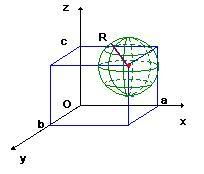# Coordinates inside a ball

• bogie

#### bogie

A ball can be defined as the inside of a sphere. It is made up of all points inside the sphere. Is there a customary way to describe the location of points in a ball, i.e. a coordinate system to define each point?

Is there a graphics program that can be used that let's you input the coordinates and visualize the defined points graphically?

Either polar or cartesian coordinates, with the origin at the center would work.

yes, we call them spherical coordinates.
where do they get these names from?! (-:

Last edited:
A ball can be defined as the inside of a sphere. It is made up of all points inside the sphere. Is there a customary way to describe the location of points in a ball, i.e. a coordinate system to define each point?

Is there a graphics program that can be used that let's you input the coordinates and visualize the defined points graphically?

The equation of a sphere, having the radius R, and center (a, b, c) is:
$$(x - a) ^ 2 + (y - b) ^ 2 + (z - c) ^ 2 = R ^ 2$$
Now, a ball is a collection of the points whose distances from the center are less than or equal to R, so, the ball has the equation:
$$(x - a) ^ 2 + (y - b) ^ 2 + (z - c) ^ 2 \leq R ^ 2$$

The equation of a sphere, having the radius R, and center (a, b, c) is:
$$(x - a) ^ 2 + (y - b) ^ 2 + (z - c) ^ 2 = R ^ 2$$
Now, a ball is a collection of the points whose distances from the center are less than or equal to R, so, the ball has the equation:
$$(x - a) ^ 2 + (y - b) ^ 2 + (z - c) ^ 2 \leq R ^ 2$$
I found this graphic: http://mathworld.wolfram.com/SphericalCoordinates.html

It is for defining points on a sphere, but I can see how the same coordinate system would be used to define the location of points in the ball.

Are your x, y and z the same as the x axis, y-axis and z axis in the graphic?

How do you’re a, b and c relate to the graphic?

(a,b,c) is the centre of the sphere, in the link youv'e given it's (0,0,0).

Instead of having the center at (0, 0, 0), my sphere has its center at (a, b, c), you can look at the attachment below. Srry, if my drawing is just so bad...The red little dot is the center having the co-ordinate (a, b, c).
The sphere is green.
Is it clearer now? :)

Last edited:
Yes. Your drawing is very good.

Using your drawing can you give me an example of how your formulas work, replacing the xyz and abc with numbers that relate to the drawing? Or do your formulas represent a general definition of a sphere and are not intended to describe a specific point on or in the sphere.

If want to try a simple programming environment, you might try writing a simple program using http://vpython.org/ and the formulas above to visualize these points in 3D.

If you don't want to write a program [which would be very instructive for you], you can try http://www.gnuplot.info/ .

Some useful interactive visualization, try this flash-based tutorial on spherical coordinates
http://mathdl.maa.org/mathDL/3/?pa=content&sa=viewDocument&nodeId=614 [Broken]

Last edited by a moderator:
If want to try a simple programming environment, you might try writing a simple program using http://vpython.org/ and the formulas above to visualize these points in 3D.

If you don't want to write a program [which would be very instructive for you], you can try http://www.gnuplot.info/ .